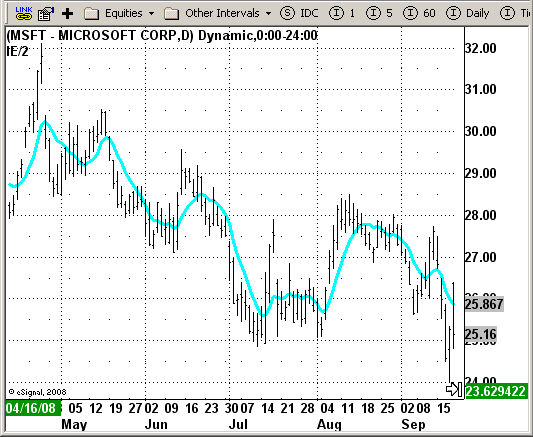# IE / 2

ICE Data Services -

File Name: IE2.efs

Description:
IE/2

Formula Parameters:

• Length: 15
• Price Data To Use: Close

Notes:
IE/2 is one of pre-studies created while T3 famous average was developing. It is calculated as

`ILRS(n)+EPMA(n))/2.`

ILRS, is an integral of linear regression slope. In this moving average, the slope of a linear regression line is simply integrated as it is fitted in a moving window of length n across the data. The derivative of ILRS is
the linear regression slope.
EPMA is an end point moving average - it is the endpoint of the linear regression line of length n as it is fitted across the data. EPMA hugs the data more closely than a simple or exponential moving average of the same length.

IE2.efsEFS Code:

```/*********************************
Provided By:
eSignal (Copyright c eSignal), a division of Interactive Data
Formula Script (EFS) is for educational purposes only and may be
modified and saved under a new file name.  eSignal is not responsible
for the functionality once modified.  eSignal reserves the right
to modify and overwrite this EFS file with each new release.

Description:
IE/2

Version:            1.0  09/23/2008

Notes:
IE/2 is one of pre-studies created while T3 famous average was developing.
It is calculated as (ILRS(n)+EPMA(n))/2.

ILRS, is an integral of linear regression slope. In this moving average,
the slope of a linear regression line is simply integrated as it is fitted
in a moving window of length n across the data. The derivative of ILRS is
the linear regression slope.

EPMA is an end point moving average - it is the endpoint of the linear regression
line of length n as it is fitted across the data. EPMA hugs the data more closely
than a simple or exponential moving average of the same length.

Formula Parameters:                     Default:
Length                                  15
Price Data To Use                      Close
**********************************/

var fpArray = new Array();
var bInit = false;

function preMain()
{
setStudyTitle("IE/2");
setCursorLabelName("IE/2");
setDefaultBarFgColor(Color.cyan, 0);
setPlotType(PLOTTYPE_LINE,0);
setDefaultBarThickness(3, 0);
setPriceStudy(true);

var x=0;
fpArray[x] = new FunctionParameter("Length", FunctionParameter.NUMBER);
with(fpArray[x++]){
setLowerLimit(1);
setDefault(15);
}

fpArray[x] = new FunctionParameter("Price", FunctionParameter.STRING);
with(fpArray[x++]){
setName("Price Data To Use");
setDefault("close");
}
}

var xIE = null;
var xMyPrice = null;

function main(Price, Length) {
var nIE = 0;

if (Price == null) Price = "close";
if (Length == null) Period = 15;

if ( bInit == false ) {
xMyPrice = eval(Price)();
xIE = efsInternal("Calc_IE", xMyPrice, Length);
bInit = true;
}

if (getCurrentBarCount() <= Length) return;

nIE = xIE.getValue(0);

return nIE;
}

function Calc_IE(xMyPrice, Length){
var nAvg = 0.0;
var nSumBars = Length * (Length - 1) * 0.5;
var nSumSqrBars = (Length - 1) * Length * (2 * Length - 1) / 6;
var nSum1 = 0.0;
var nSumY = 0.0;

for (var i = 0; i < Length; i++)
{
nAvg += xMyPrice.getValue(-i);
nSum1 += i * xMyPrice.getValue(-i);
nSumY += xMyPrice.getValue(-i);
}

nAvg /= Length;

var nSum2 = nSumBars * nSumY;
var nNum1 = Length * nSum1 - nSum2;
var nNum2 = nSumBars * nSumBars - Length * nSumSqrBars;
var nLinRegSlope = 0.0;

if (nNum2 != 0)
{
nLinRegSlope = nNum1 / nNum2;
}

var nILRS = nLinRegSlope + nAvg;
var nIntercept = (nSumY - nLinRegSlope * nSumBars) / Length;
var nLinearRegValue = nIntercept + nLinRegSlope * (Length - 1);
var nIE =  (nILRS + nLinearRegValue) / 2;

return nIE;
}```